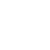PV_SpectralEnhance:
Filter:
Classes (extension) |

# PV_SpectralEnhance : PV_ChainUGen : WidthFirstUGen : UGen : AbstractFunction : ObjectExtension

A harmonic enhancer
Source: JoshPV.sc

## Class Methods

### PV_SpectralEnhance.new(buffer, numPartials: 8, ratio: 2, strength: 0.1)

#### Arguments:

 buffer The FFT buffer to filter. numPartials The number of partials above each bin to add magnitude to. ratio The ratio between the original partial and the partials above to add harmonic content to (should be greater the 1... usually 2 or greater). strength A multiplier for each successive partial.NOTE: How numPartials, ratio and strength are used. If you have a bin (e.g., bin 10) with magnitude of 1, magnitude will be added to numPartial bins above that bin according to the ratio. If numPartial is 4 and the ratio is 2, magnitude will be added to bins 20, 30, 40 and 50. If strength is 0.1, bin 20 will get an additional 0.1 * the original magnitude (0.1), bin 30 will get (0.1 / 2), bin 40 will get (0.1 / 3) and bin 50 (0.1 / 4). Strength can be any positive number.